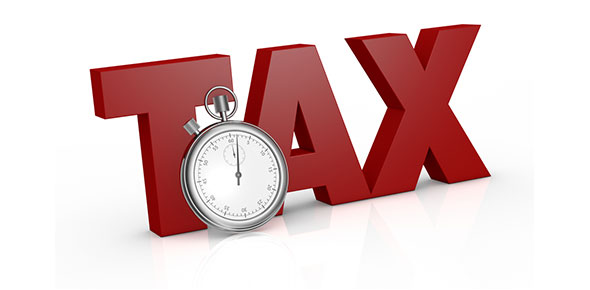# Do You Know Where Your Taxes Are Going?

13 Questions | Total Attempts: 47SettingsOn April 15, 2013, millions of Americans will file their income taxes for 2012. Find out where your federal income taxes went during Fiscal Year 2012. Note: This quiz looks only at federal income taxes, not payroll taxes.

• 1.
How many cents of each income tax dollar went towards food and agriculture?
• A.

4.9 cents

• B.

4.4 cents

• C.

3.8 cents

• D.

5.2 cents

• 2.
How many cents of each income tax dollar went towards transportation?
• A.

1.4 cents

• B.

2.3 cents

• C.

1.1 cents

• D.

2.8 cents

• 3.
How many cents of each income tax dollar went towards health care?
• A.

22.3 cents

• B.

26.8 cents

• C.

20.0 cents

• D.

15.7 cents

• 4.
How many cents of each income tax dollar went towards science?
• A.

2.5 cents

• B.

1.0 cents

• C.

3.2 cents

• D.

8.4 cents

• 5.
How many cents of each income tax dollar went towards government?
• A.

15.8 cents

• B.

4.6 cents

• C.

7.9 cents

• D.

5.4 cents

• 6.
How many cents of each income tax dollar went towards military?
• A.

5.8 cents

• B.

26.5 cents

• C.

13.2 cents

• D.

9.7 cents

• 7.
How many cents of each income tax dollar went towards international affairs?
• A.

30.2 cents

• B.

1.4 cents

• C.

8.9 cents

• D.

19.3 cents

• 8.
How many cents of each income tax dollar went towards education?
• A.

22.6 cents

• B.

14.3 cents

• C.

3.5 cents

• D.

5.9 cents

• 9.
How many cents of each income tax dollar went towards interest on federal debt?
• A.

13.6 cents

• B.

23.8 cents

• C.

6.8 cents

• D.

33.1 cents

• 10.
How many cents of each income tax dollar went towards energy and environment?
• A.

18.2 cents

• B.

9.4 cents

• C.

2.8 cents

• D.

2.1 cents

• 11.
How many cents of each income tax dollar went towards unemployment and labor?
• A.

4.3 cents

• B.

8.5 cents

• C.

12.2 cents

• D.

28.4 cents

• 12.
How many cents of each income tax dollar went towards veterans benefits?
• A.

4.3 cents

• B.

9.2 cents

• C.

19.3 cents

• D.

22.6 cents

• 13.
How many cents of each income tax dollar went towards housing and community?
• A.

3.1 cents

• B.

4.2 cents

• C.

8.6 cents

• D.

12.3 cents

Related TopicsBack to top